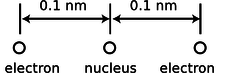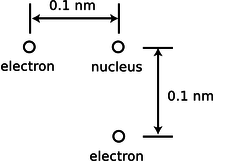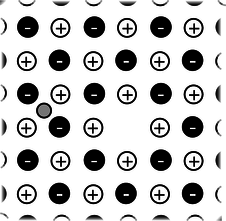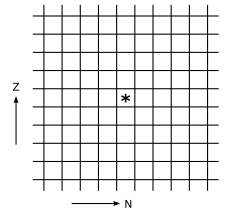$$\require{cancel}$$

# Problems

•• Contributed by Benjamin Crowell
• Professor (Physics) at Fullerton Collegea / Problem 1. Top: A realistic picture of a neuron. Bottom: A simplified diagram of one segment of the tail (axon).

1. The figure shows a neuron, which is the type of cell your nerves are made of. Neurons serve to transmit sensory information to the brain, and commands from the brain to the muscles. All this data is transmitted electrically, but even when the cell is resting and not transmitting any information, there is a layer of negative electrical charge on the inside of the cell membrane, and a layer of positive charge just outside it. This charge is in the form of various ions dissolved in the interior and exterior fluids. Why would the negative charge remain plastered against the inside surface of the membrane, and likewise why does not the positive charge wander away from the outside surface?

2. The Earth and Moon are bound together by gravity. If, instead, the force of attraction were the result of each having a charge of the same magnitude but opposite in sign, find the quantity of charge that would have to be placed on each to produce the required force.(answer check available at lightandmatter.com)b / Problem 3.

3. A helium atom finds itself momentarily in this arrangement. Find the direction and magnitude of the force acting on the right-hand electron. The two protons in the nucleus are so close together ($$\sim1$$ fm) that you can consider them as being right on top of each other.

4. $$^{234}\text{Pu}$$ decays either by electron decay or by alpha decay. (A given $$^{234}\text{Pu}$$ nucleus may do either one; it's random.) What are the isotopes created as products of these two modes of decay?

5. Suppose that a proton in a lead nucleus wanders out to the surface of the nucleus, and experiences a strong nuclear force of about 8 kN from the nearby neutrons and protons pulling it back in. Compare this numerically to the repulsive electrical force from the other protons, and verify that the net force is attractive. A lead nucleus is very nearly spherical, is about 6.5 fm in radius, and contains 82 protons, each with a charge of $$+e$$, where $$e=1.60\times10^{-19}\ \text{C}$$. (answer check available at lightandmatter.com)

6. The nuclear process of beta decay by electron capture is described parenthetically on page 493. The reaction is $$\text{p}+\text{e}^- \rightarrow \text{n}+\nu$$.
(a) Show that charge is conserved in this reaction.
(b) Conversion between energy and mass is discussed in chapter 7. Based on these ideas, explain why electron capture does not occur in hydrogen atoms. (If it did, matter wouldn't exist!)c / Problem 7.

7. In the semifinals of an electrostatic croquet tournament, Jessica hits her positively charged ball, sending it across the playing field, rolling to the left along the $$x$$ axis. It is repelled by two other positive charges. These two charges are fixed on the $$y$$ axis at the locations shown in the figure. The two fixed charges are equal to each other, but not to the charge of the ball.
(a) Express the force on the ball in terms of the ball's position, $$x$$.
(b) At what value of $$x$$ does the ball experience the greatest deceleration? Express you answer in terms of $$b$$. (Assume the ball has enough energy to continue through, so you don't have to worry about whether it actually gets as far as this particular value of $$x$$.) (Based on a problem by Halliday and Resnick.)

8. Suppose that at some instant in time, a wire extending from $$x=0$$ to $$x=\infty$$ holds a charge density, in units of coulombs per meter, given by $$ae^{-bx}$$. This type of charge density, $$dq/dx$$, is typically notated as $$\lambda$$ (Greek letter lambda). Find the total charge on the wire.(answer check available at lightandmatter.com)

9. Use the nutritional information on some packaged food to make an order-of-magnitude estimate of the amount of chemical energy stored in one atom of food, in units of joules. Assume that a typical atom has a mass of $$10^{-26}$$ kg. This constitutes a rough estimate of the amounts of energy there are on the atomic scale. [See chapter 0 for help on how to do order-of-magnitude estimates. Note that a nutritional “calorie” is really a kilocalorie; see page 943.](answer check available at lightandmatter.com)d / Problem 10.

10. The helium atom of problem 3 has some new experiences, goes through some life changes, and later on finds itself in the configuration shown here. What are the direction and magnitude of the force acting on the bottom electron? (Draw a sketch to make clear the definition you are using for the angle that gives direction.)(answer check available at lightandmatter.com)

11. A neon light consists of a long glass tube full of neon, with metal caps on the ends. Positive charge is placed on one end of the tube, and negative charge on the other. The electric forces generated can be strong enough to strip electrons off of a certain number of neon atoms. Assume for simplicity that only one electron is ever stripped off of any neon atom. When an electron is stripped off of an atom, both the electron and the neon atom (now an ion) have electric charge, and they are accelerated by the forces exerted by the charged ends of the tube. (They do not feel any significant forces from the other ions and electrons within the tube, because only a tiny minority of neon atoms ever gets ionized.) Light is finally produced when ions are reunited with electrons. Give a numerical comparison of the magnitudes and directions of the accelerations of the electrons and ions. [You may need some data from appendix 5.] (answer check available at lightandmatter.com)

12. The subatomic particles called muons behave exactly like electrons, except that a muon's mass is greater by a factor of 206.77. Muons are continually bombarding the Earth as part of the stream of particles from space known as cosmic rays. When a muon strikes an atom, it can displace one of its electrons. If the atom happens to be a hydrogen atom, then the muon takes up an orbit that is on the average 206.77 times closer to the proton than the orbit of the ejected electron. How many times greater is the electric force experienced by the muon than that previously felt by the electron?(answer check available at lightandmatter.com)

13. (a) Recall that the gravitational energy of two gravitationally interacting spheres is given by $$U_g=-Gm_1m_2/r$$, where $$r$$ is the center-to-center distance. What would be the analogous equation for two electrically interacting spheres? Justify your choice of a plus or minus sign on physical grounds, considering attraction and repulsion.(answer check available at lightandmatter.com)
(b) Use this expression to estimate the energy required to pull apart a raisin-cookie atom of the one-electron type, assuming a radius of $$10^{-10}$$ m.(answer check available at lightandmatter.com)
(c) Compare this with the result of problem 9.

14. If you put two hydrogen atoms near each other, they will feel an attractive force, and they will pull together to form a molecule. (Molecules consisting of two hydrogen atoms are the normal form of hydrogen gas.) Why do they feel a force if they are near each other, since each is electrically neutral? Shouldn't the attractive and repulsive forces all cancel out exactly? Use the raisin cookie model. (Students who have taken chemistry often try to use fancier models to explain this, but if you cannot explain it using a simple model, you probably don't understand the fancy model as well as you thought you did!)e / Problem 15.

15. The figure shows one layer of the three-dimensional structure of a salt crystal. The atoms extend much farther off in all directions, but only a six-by-six square is shown here. The larger circles are the chlorine ions, which have charges of $$-e$$. The smaller circles are sodium ions, with charges of $$+e$$. The distance between neighboring ions is about 0.3 nm. Real crystals are never perfect, and the crystal shown here has two defects: a missing atom at one location, and an extra lithium atom, shown as a grey circle, inserted in one of the small gaps. If the lithium atom has a charge of $$+e$$, what is the direction and magnitude of the total force on it? Assume there are no other defects nearby in the crystal besides the two shown here. \hwhint{hwhint:nacl}(answer check available at lightandmatter.com)

16. [See section 0.2 for help on how to do order-of-magnitude estimates.] Suppose you are holding your hands in front of you, 10 cm apart.
(a) Estimate the total number of electrons in each hand.
(b) Estimate the total repulsive force of all the electrons in one hand on all the electrons in the other.
(c) Why don't you feel your hands repelling each other?
(d) Estimate how much the charge of a proton could differ in magnitude from the charge of an electron without creating a noticeable force between your hands.

# Exercises

## Exercise A: Nuclear decay

1. Consulting a periodic table, find the $$N$$, $$Z$$, and $$A$$ of the following:

 N Z A 4He 244Pu

2. Consider the following five decay processes:

• $$\alpha$$ decay
• $$\gamma$$ decay
• $$\text{p} \rightarrow \text{n} + \text{e}^+ + \nu$$ ($$\beta^+$$ decay)
• $$\text{n} \rightarrow \text{p} + \text{e}^- + \bar{\nu}$$ ($$\beta^-$$ decay)
• $$\text{p} + \text{e}^- \rightarrow \text{n} + \nu$$ (electron capture)

What would be the action of each of these on the chart of the nuclei? The * represents the original nucleus.3. (a) Suppose that $${}^{244}\text{Pu}$$ undergoes perfectly symmetric fission, and also emits two neutrons. Find the daughter isotope.

(b) Is the daughter stable, or is it neutron-rich or -poor relative to the line of stability? (To estimate what's stable, you can consult a periodic table and use the average atomic mass as an approximation ot the stable value of $$A$$.)

(c) Consulting the chart of the nuclei (fig. s on p. 498), explain why it turns out this way.

(d) If the daughter is unstable, which process from question #2 would you expect it to decay by?

(c) 1998-2013 Benjamin Crowell, licensed under the Creative Commons Attribution-ShareAlike license. Photo credits are given at the end of the Adobe Acrobat version.

## Contributors

Benjamin Crowell (Fullerton College). Conceptual Physics is copyrighted with a CC-BY-SA license.